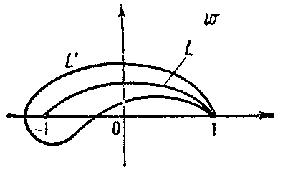# Zhukovskii function

The rational function

$$w=\lambda(z)=\frac12\left(z+\frac1z\right)$$

of the complex variable $z$. It is important for its applications in fluid mechanics, which were discovered by N.E. Zhukovskii (see , ), particularly in constructing and studying the Zhukovskii profile (Zhukovskii wing). Suppose that a circle $K$ is given in the $z$-plane passing through the points $z=\pm1$ (Fig. a), together with a circle $K'$ touching $K$ on the outside at $z=1$, with centre $\alpha$ and radius $\rho$. Under the mapping $w=\lambda(z)$, the image of $K'$ is a closed curve $L'$ with a cusp at the point $w=1$, touching an arc of the circle $L$ (the image of $K$) at that point; this image is represented in Fig. band is the Zhukovskii profile.Figure: z099280aFigure: z099280b

The function $w=\lambda(\rho t+\alpha)$ maps the exterior of the unit circle in the $t$-plane to the exterior of $L'$. To obtain a Zhukovskii profile of a more general shape and disposition, the generalized Zhukovskii function is applied (see , , ):

$$w=\frac12(a-b)z+\frac12(a+b)\frac1z,\quad a>b>0.$$

How to Cite This Entry:
Zhukovskii function. Encyclopedia of Mathematics. URL: http://encyclopediaofmath.org/index.php?title=Zhukovskii_function&oldid=34330
This article was adapted from an original article by E.D. Solomentsev (originator), which appeared in Encyclopedia of Mathematics - ISBN 1402006098. See original article